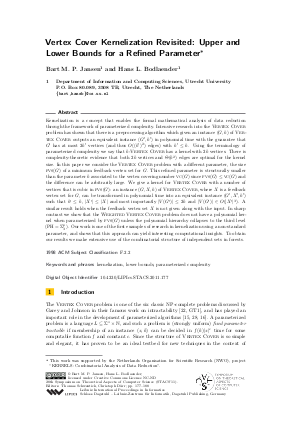Document# Vertex Cover Kernelization Revisited: Upper and Lower Bounds for a Refined Parameter

### Authors Bart M. P. Jansen, Hans L. Bodlaender## File

LIPIcs.STACS.2011.177.pdf
• Filesize: 0.63 MB
• 12 pages

## Cite As

Bart M. P. Jansen and Hans L. Bodlaender. Vertex Cover Kernelization Revisited: Upper and Lower Bounds for a Refined Parameter. In 28th International Symposium on Theoretical Aspects of Computer Science (STACS 2011). Leibniz International Proceedings in Informatics (LIPIcs), Volume 9, pp. 177-188, Schloss Dagstuhl - Leibniz-Zentrum für Informatik (2011)
https://doi.org/10.4230/LIPIcs.STACS.2011.177

## Abstract

Kernelization is a concept that enables the formal mathematical analysis of data reduction through the framework of parameterized complexity. Intensive research into the Vertex Cover problem has shown that there is a preprocessing algorithm which given an instance (G,k) of Vertex Cover outputs an equivalent instance (G',k') in polynomial time with the guarantee that G' has at most 2k' vertices (and thus O((k')^2) edges) with k' <= k. Using the terminology of parameterized complexity we say that k-Vertex Cover has a kernel with 2k vertices. There is complexity-theoretic evidence that both 2k vertices and Theta(k^2) edges are optimal for the kernel size. In this paper we consider the Vertex Cover problem with a different parameter, the size fvs(G) of a minimum feedback vertex set for G. This refined parameter is structurally smaller than the parameter k associated to the vertex covering number VC(G) since fvs(G) <= VC(G) and the difference can be arbitrarily large. We give a kernel for Vertex Cover with a number of vertices that is cubic in fvs(G): an instance (G,X,k) of Vertex Cover, where X is a feedback vertex set for G, can be transformed in polynomial time into an equivalent instance (G',X',k') such that k' <= k, |X'| <= |X| and most importantly |V(G')| <= 2k and |V(G')| in O(|X'|^3). A similar result holds when the feedback vertex set X is not given along with the input. In sharp contrast we show that the Weighted Vertex Cover problem does not have polynomial kernel when parameterized by fvs(G) unless the polynomial hierarchy collapses to the third level (PH=Sigma_3^p). Our work is one of the first examples of research in kernelization using a non-standard parameter, and shows that this approach can yield interesting computational insights. To obtain our results we make extensive use of the combinatorial structure of independent sets in forests.
##### Keywords
• kernelization
• lower bounds
• parameterized complexity

## Metrics

• Access Statistics
• Total Accesses (updated on a weekly basis)
0